# hi i need answer from part d Question 2 (48 marks) Consider a firm which produces a good, y, using two factors of...

hi i need answer from part d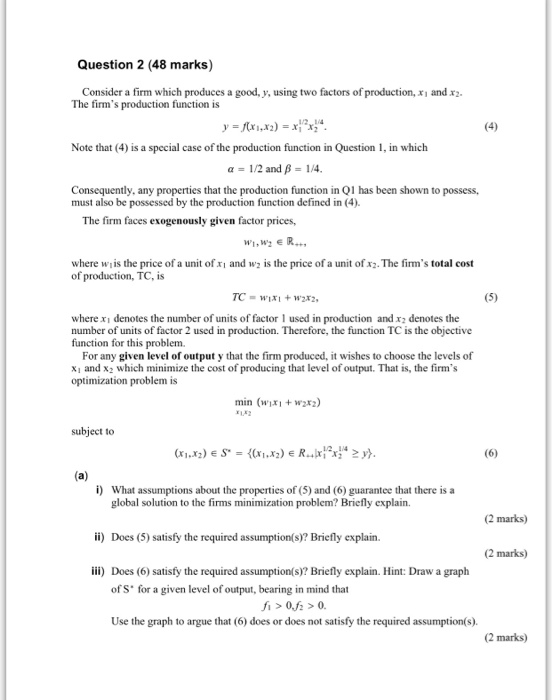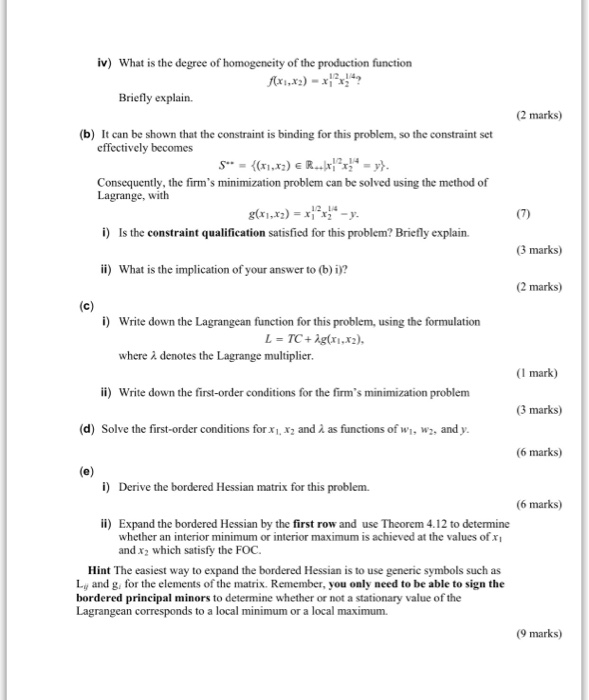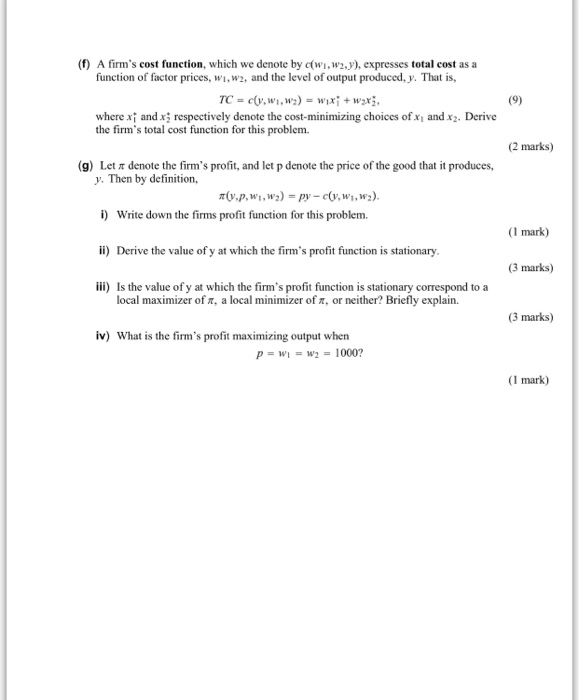Question 2 (48 marks) Consider a firm which produces a good, y, using two factors of production, xi and x2 The firm's production function is Note that (4) is a special case of the production function in Question 1, in which α-1/2 and β-14. Consequently, any properties that the production function in Q1 has been shown to possess, must also be possessed by the production function defined in (4). The firm faces exogenously given factor prices, where w is the price of a unit of xi and w is the price of a unit of x2. The firm's total cost of production, TC, is where xi denotes the number of units of factor 1 used in production and x2 denotes the number of units of factor 2 used in production. Therefore, the function TC is the objective function for this problem. For any given level of output y that the firm produced, it wishes to choose the levels of x1 and x2 which minimize the cost of producing that level of output. That is, the firm's optimization problem is min (wx +w2xz) subject to i) What assumptions about the properties of (5) and (6) guarantee that there is a global solution to the firms minimization problem? Briefly explain. (2 marks) ii) Does (5) satisfy the required assumption(s)? Briefly explain. (2 marks) ili) Does (6) satisfy the required assumption(s)? Briefly explain. Hint: Draw a graph of S' for a given level of output, bearing in mind that 0.f0. Use the graph to argue that (6) does or does not satisfy the required assumption(s) (2 marks)
iv) What is the degree of ty of the production function Briefly explain. (2 marks) (b) It can be shown that the constraint is binding for this problem, so the constraint set effectively becomes Consequently, the firm's minimization problem can be solved using the method of Lagrange, with 12 14 i) Is the constraint qualification satisfied for this problem? Briefly explain (3 marks) i) What is the implication of your answer to (b) i? (2 marks) i) Write down the Lagrangean function for this problem, using the formulation where λ denotes the Lagrange multiplier (1 mark) i) Write down the first-order conditions for the firm's minimization problem (3 marks) (d) Solve the first-order conditions for x. x2 and λ as functions of wl, w2, and y. 6 marks) i) Derive the bordered Hessian matrix for this problem. (6 marks) ii) Expand the bordered Hessian by the first row and use Theorem 4.12 to determine whether an interior minimum or interior maximum is achieved at the values of xI and x2 which satisfy the FOC. Hint The easiest way to expand the bordered Hessian is to use generic symbols such as Liy and g, for the elements of the matrix. Remember, you only need to be able to sign the bordered principal minors to determine whether or not a stationary value of the Lagrangean corresponds to a local minimum or a local maximum. (9 marks)
(f) A firm's cost function, which we denote by c(wi, w2y), expresses total cost as a function of factor prices, wi,w2, and the level of output produced, y. That is where xi and x respectively denote the cost-minimizing choices of xi and xz. Derive the firm's total cost function for this problem. (2 marks) (g) Let π denote the firm's profit, and let p denote the price of the good that it produces, y. Then by definition Write down the firms profit function for this problem. (I mark) ii) Derive the value of y at which the firm's profit function is stationary 3 marks) iii) Is the value of y at which the firm's profit function is stationary correspond to a local maximizer of π, a local minimizer or, or neither? Briefly explain. 3 marks) iv) What is the firm's profit maximizing output when (I mark)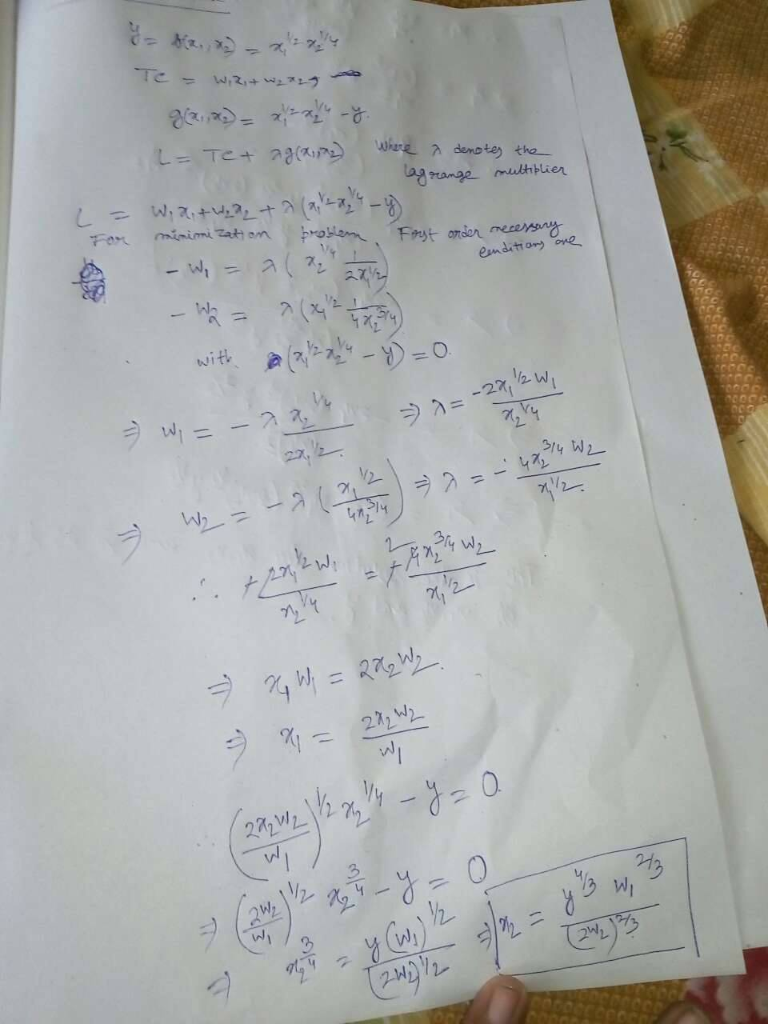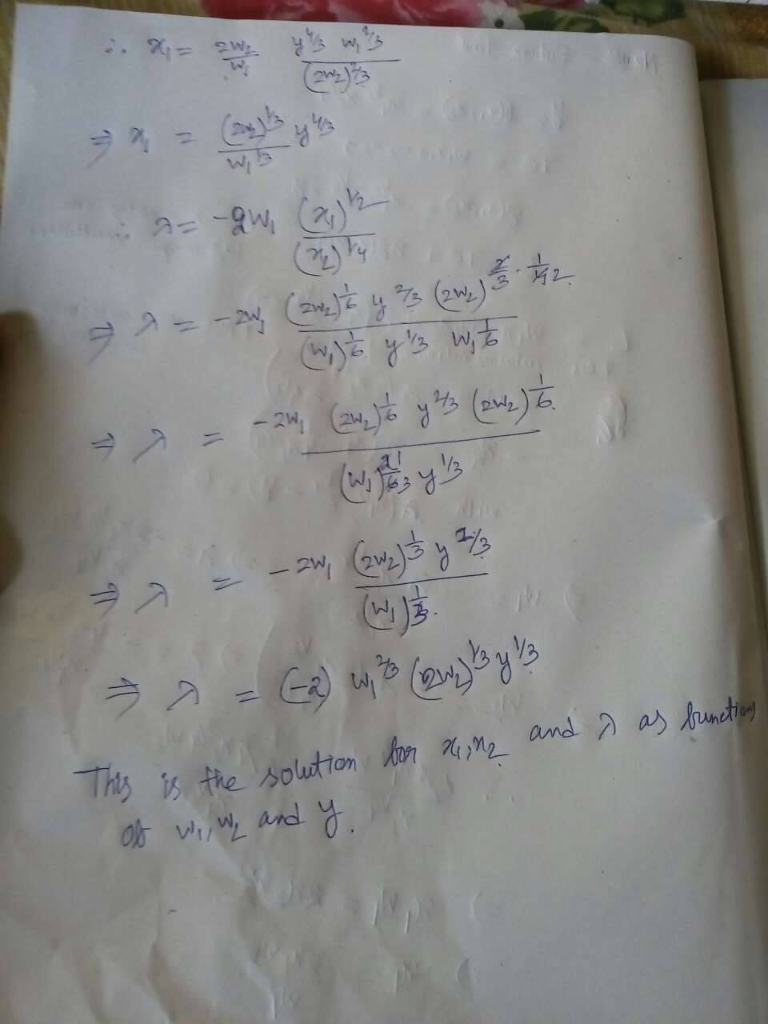##### Add Answer of: hi i need answer from part d Question 2 (48 marks) Consider a firm which produces a good, y, using two factors of...
More Homework Help Questions Additional questions in this topic.